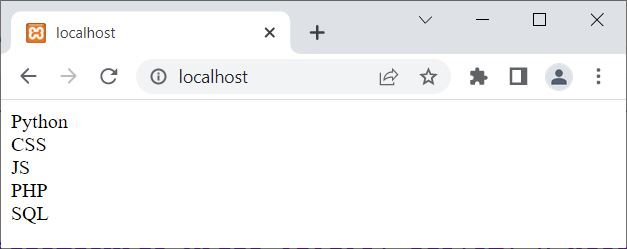# foreach loop in PHP with examples

Unlike other loops, the "foreach" loop in PHP works only for arrays. The foreach loop is used when we need to execute a code blocks multiple times for each element of an array.

## PHP foreach loop syntax

The syntax of the foreach loop in PHP is:

```foreach(\$array as \$value)
{
// block of code;
}```

For the first time, the first element of the array gets assigned to \$value. Similarly, at the second time, the second element of the array gets assigned to \$value, and so on. This process continues until the last element. For example:

```<?php
\$languages = array("Python", "CSS", "JS", "PHP", "SQL");
foreach(\$languages as \$x)
{
echo \$x;
echo "<BR>";
}
?>```

Five strings, each of which represents a different programming language, are used to initialize the array \$languages in the PHP code above.

The \$languages array's elements are then iterated over using a foreach loop. The code creates a variable called \$x and sets it to the value of the current element while it is in the loop.

The echo statement is then used to echo the value of \$x, followed by a line break. Each programming language will appear on a separate line as a result.

The foreach loop iterates until all of the elements in the \$languages array have been processed.

The output of the above PHP example is shown in the snapshot given below:## PHP foreach loop example

This example uses an array whose elements are key-value pairs.

```<?php
echo "\$k = \$v <BR>";
?>```

The PHP code above creates three key-value pairs for the \$addr associative array. The corresponding value for each key contains the specific information for that key. Each key represents a piece of information about a person, such as their name, age, and city of residence.

Then, it iterates through each key-value pair in the \$addr array using a foreach loop. The code assigns the current value to a variable called \$v and the current key to a variable called \$k within the loop.

The echo statement is then used to echo the current key-value pair with string interpolation ("\$k = \$v") and a line break ("<BR>"). Each key-value pair will be displayed separately as a result.

The foreach loop iterates until all of the key-value pairs in the \$addr array have been processed.

The snapshot given below shows the sample output produced by this PHP example, on foreach loop:### Advantages of the foreach loop in PHP

• "foreach" loops are a good option for beginners or for straightforward tasks because they are simple to use and comprehend.
• Because "foreach" loops have clear and simple syntax, your code will be more effective and less prone to mistakes.
• When iterating over arrays or other iterable data structures, particularly large ones, "foreach" loops are typically more effective than classic "for" loops.

### Disadvantages of the foreach loop in PHP

• For some tasks, "foreach" loops may not be the best choice because they do not give you as much control over the loop index or iteration as other loop types do.
• If you alter the array or data structure while the loop is running, it's possible for errors or unexpected outcomes to result from the unpredictable behavior of the loop.
• When iterating over arrays with numerical indexes, "foreach" loops are less effective than traditional "for" loops because the former needs extra variables to store the current key and value.

PHP Online Test

« Previous Tutorial Next Tutorial »